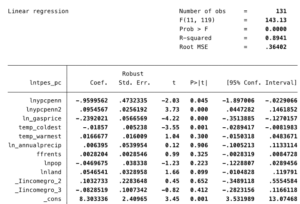# BUSM 4554: Basic Econometrics QUESTION 1) We model the salary of the average inhabitant of Pinkland. Salaries are a function of education (expressed in years), working hours (expressed in hours), firm sales and sector. There are 3 sectors in Pinkland, namely agriculture, industry and service

QUESTION 1)

We model the salary of the average inhabitant of Pinkland. Salaries are a function of education (expressed in years), working hours (expressed in hours), firm sales and sector. There are 3 sectors in Pinkland, namely agriculture, industry and service.

Salaries are expressed in pinkies (currency of Pinkland). The model is estimated as follows:

Log(salary) = 1.2+  0.08*educ+ 0.6*log(hours) + 0.16*log(sales) + 0.37*industry +0.56*service N=800

R2 = 0.78

• Interpret the coefficient on education. What type of relationship is this?
• Interpret the coefficient on “hours” and “sales”. What type of relationship are these?
• We have 3 categorical variables in the regression. Which is the base category?
• Interpret the coefficient on “industry” and “service”.
• Interpret the R2. Is this high enough in your opinion?

Don’t forget: Log referrers to natural logarithm!

QUESTION  2

We model energy use per capita of 131 countries in a given year as follows:

Lntpes_pc =

The variables are defined as follows:

Lntpes_pc = log of total primary energy consumption per capita (ktoe)

Lnypcpenn =log of GDP per capita (USD)

Lnypcpenn2 = square of log of GDP per capita (USD)

Ln_gasprice = log of pump price for gasoline (USD/liter)

LnAnnualprecip= log of annual precipitation (mm)

Temp_coldest = average temperature for the coldest month in a year (in C)

Temp_warmest= average temperature for the warmest month in a year (in C)

ffrents = Fossil Fuel Rents (% of GDP)

LnPop = log of population (in millions)

LnLand = log of land area (in km2)

I_Incomegroup = refers to income groups “1” , “2” and “3”, low, mid and high income countries.

*” Log” always refers to natural logs or “ln” here.Log referrers to natural logarithm!

• Interpret the constant and its p-value.
• Interpret the coefficient on gas price and its p-value.
• Interpret the coefficient on annual precipitation and carry out a t-test to determine the significance of the coefficient.
• Interpret the coefficient on population and its p-value.
• The above model belongs to the class of nonlinear equations. Calculate the turning point of the nonlinear relationship.
• Is this a U-shaped or inverted U-shaped relationship?
• Analyse the relationship between energy use per capita and GDP pc based on these insights. How does energy use per capita change with GDPpc? Prepare a graph!
• Please define MLR 2 and describe if MLR 2 is likely to hold or not?
• Please define MLR 4 and describe if MLR 4 is likely to hold or not?

Critical values for the standard normal distribution (z)

 Confidence level  (1-α) Level of Significance  (α) Two-SidedCritical Valuecα/2 One-Sided,Upper-TailCritical Valuecα One-Sided,Lower-TailCritical Value-cα 90% 10% 1.645 1.28 -1.28 95% 5% 1.96 1.645 -1.645 99% 1% 2.58 2.33 -2.33